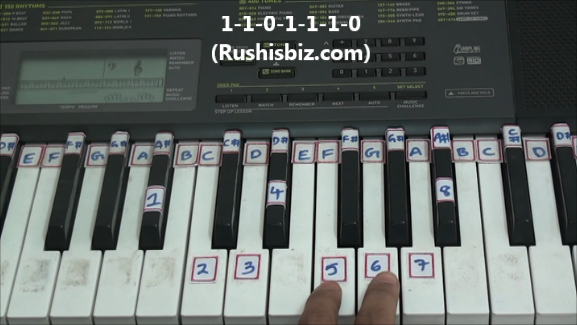HINDI - A B C D E F G H I J K L M N O P Q R S T U V W X Y Z

TAMIL - A B C D E F G H I J K L M N O P Q R S T U V W X Y Z

TELUGU - A B C D E F G H I J K L M N O P Q R S T U V W X Y Z

# “A#/Bb” Major Scale Positions – Piano Tutorials

Notes:  A#-1, C-2, D-3, D#-4, F-5, G-6, A-7, A#-8

Since it is a ‘A#’ major scale obviously ‘A#’ will be first note, how to find the remaining notes ?

The simple formula which is used for any major scale:

One- One- Zero- One-One- One-Zero  (1-1-0-1-1-1-0)

What do you mean by Ones and Zeros ?

One’s (whole step) – If there is a one key between two notes, then the interval of those two notes is One.

Eg: from A# to C the interval is “one key”

Zero’s (half step) – If there are no keys or zero keys between two notes, then the interval of those two notes is Zero

Eg: from D to D# the interval is “zero” (Immediate keys)‘A#’ Major Scale Positions – Video Tutorials